Last updated 2018-06-20 by Muchen He (44638154)

# Assignment 2

## Problem 1

### Description

At an 8% interest rate, if you were to invest $10,000 into one of the following investments, which one should you choose? Which factors (e.g. Single Payment Compound Amount Factor) would you use to evaluate each investment?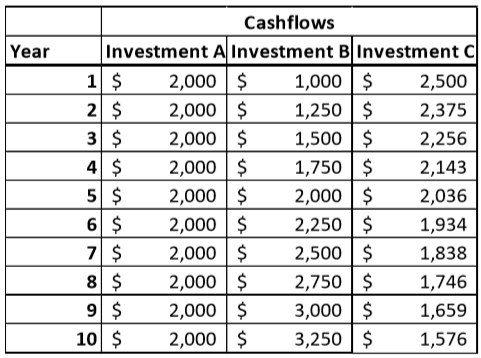If you instead decided to donate the$10,000 to an endowment fund for a charity you favour, how much annual revenue would the charity receive from your gift, assuming the same 8% interest? What would the Future Value of the endowment be?

### Analysis

Investment A is a uniforms series because of its uniform cashflows. Investment B is arithmetic series as the cash flow increases linearly at $250 each period. Investment C is a geometric series except the growth is actually negative. Investment C's growth is -5%. Investment A For investment A, we look at its uniform series present worth factor. We use the formula with $A=2000$ and $i=0.08$. Investment B For investment B, we will utilize arithmetic gradient uniform series factor to turn the periodically increasing cashflow into an equivalent cashflow. Let $A=1000$ be the uniform cashflow (since the first cashflow is$1000). Let $G=250$. Then we use the formula to get equivalent annuity:

Then apply the same method used for investment A using the equivalent arithmetic gradient uniform series factor to find the present worth factor. Except we include the original uniform cash flow as well as the equivalent cash flow of the arithmetic series:

Investment C

This is a geometric series with growth rate $g=-0.05$. Because $i\neq g$, we apply the present worth factor formula:

## Problem 2

Problem 2 Excel sheet and scrap work available here.

### Description

SAN Swiss Arms is a Switzerland based manufacturing company that makes firearms. Swiss Arms is considering manufacturing a rifle for sale in Canada. Canada is a small market for their product, but they have an existing design they could use. However, it would need to be modified to meet Canadian firearms legislation. The engineering and testing work for the design changes are estimated to be 875,000€.

Swiss Arms estimates they will sell 1,000 rifles in the first year of production, increasing linearly to 1,450 rifles in the 10th year. The rifles sell for 1,425€ each.

Rifle production can be done on an existing production line, except the new design requires one process to be modified. This can be done manually, which would incur no capital costs and give a cost per rifle of 1,150€. Alternatively, Swiss Arms can purchase a new tool for the process which would cost 240,000€. It will give a cost per rife of 1,060€, but the tool is only good for four years and has no salvage value.

Evaluate the alternatives using both NPV and EACF. If Swiss Arms considers 13.5% to be its interest rate, should they proceed with the project? If so, which alternative should they choose?

Bonus Questions: SAN Swiss Arms rifle have been involved in some legal/political controversy in Canada over the last few years. What kind of things can Swiss Arms do in this analysis to account for this risk?

### Analysis

Note: NPV stands for net present value. EACF stands for equivalent annuity cashflow.

First, our gathered known variables are as follows:

Let's assume that the growth of sales per unit increases linearly from 1000 to 1450 per year. Since the variable cost and revenue is uniform over time, this forms a linearly increasing cashflow. Thus, we will use the arithmetic series to model.

#### Economic Criteria

We notice that our input is not fixed as we can have varying costs. But our output is somewhat fixed, because we need to meet the requirements of the estimated sale volume.

Thus, in NPV analysis, our criteria is to minimize the present worth cost and maximize the net present value.

In EACF analysis we want to minimize the equivalent uniform annual cost (EUAC).

#### Strategies

The tools last 4 years but our analysis period is 10 years. We assume that the tools are repeatable purchases. There are a number of permutations on which when the tools can be bought. To keep the analysis simpler, let's assume that we can purchase the tools not once, once, twice, or three times; all consecutively after the last tool set fails starting at the beginning of year 1.

This leaves us with 4 main alternatives:

2. Buy tools at the beginning of year 1 (end of year 0)
3. Buy tools at the beginning of year 1 and 5
4. Buy tools at the beginning of year 1, 5, and 9

#### Number Crunching

##### Strategy 1

For strategy 1, we compute the cost and revenue for each year and convert it to present worth using single payment present worth factor on each year, which is given as $(1+i)^{-n}$.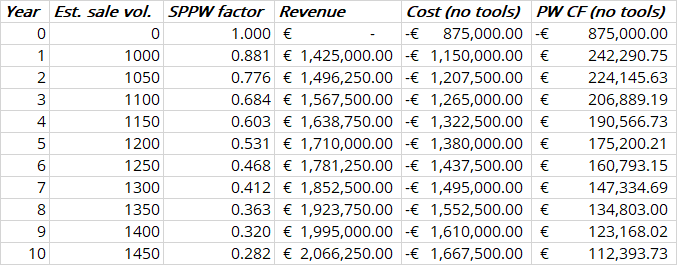The sum of all the present worth cash flows for each year gives us the NPW: $\boxed{\euro 848,585}$.

We compute the yearly costs and benefits given that the net income for each engineer is $8000 each. Below is the table of calculations and the cashflow diagram.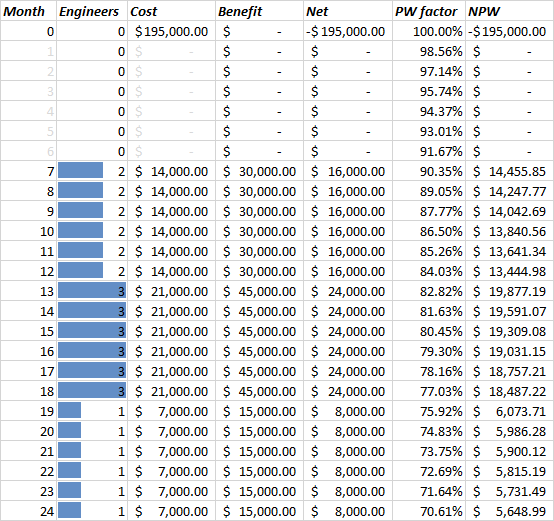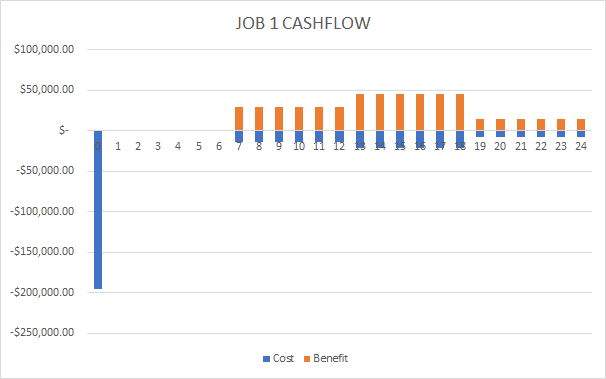Using the definition for internal rate of return calculation, where present worth costs equal to present worth benefits (check the excel sheet for more details in calculation): We isolate and solve for $i$ we get our equivalent monthly nominal interest rate. The nominal annual interest rate is then $i\times 12$. Then formula $i_a=(1+i)^m-1$ we obtain the effective interest rate, where $m$ is 12 months in a year. Finally, we get IRR of $\boxed{38.939\%}$. This is more than the MARR of 19% which indicate that this could a viable solution. Furthermore, we can plot the NPV vs. effective interest rate graph: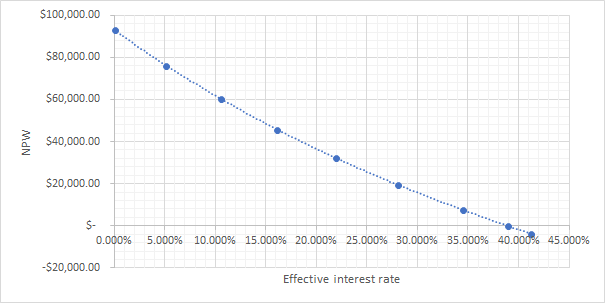#### Job 2 Similarly with job 1, we compute the annual cashflow with the bidding price of$535k. However, there are additional costs for construction: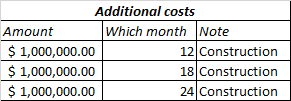Along with, the government also reimburses the construction costs with 10% profit margin. This means that for $1M we spend for construction, the government will pay $\1\text{M}\div (1-0.1)=\1.\bar 1\text M$. Because the government will hold on to 20%, they will only pay $0.8\times \1.\bar 1 \text M=0.\bar 8\text M$ and the rest at the end: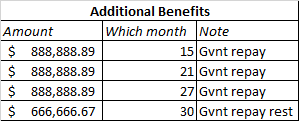For more details, consult the spreadsheet. The final cashflow diagram resembles as follows: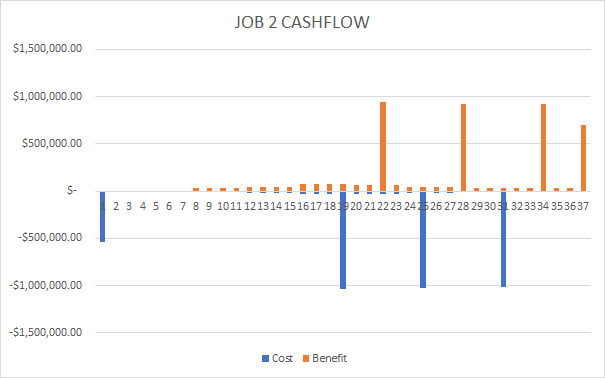The exact same method for computing IRR is applied here and we get IRR of $\boxed{23.87\%}$ which is lower than that of job 1, but still greater than the MARR. The NPV vs. effective interest rate graph is as follows: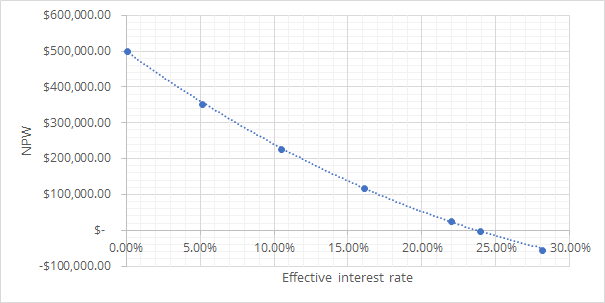#### Incremental Analysis To compare the two alternatives, I assume that these projects can be purchased repeatedly. But each time purchased, a new proposal (along with the 6 month period) is required. This enables a relatively easier analysis period, which is the LCM of 24 months and 36 months which is 72 months. Since the initial cost for job 2 is greater, we will take the difference of (cost of job 2 minus cost of job 1).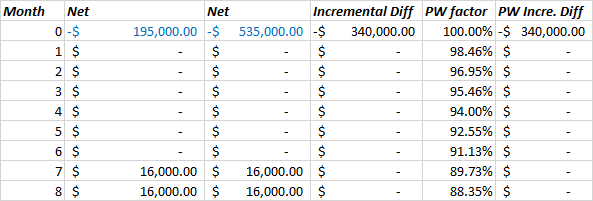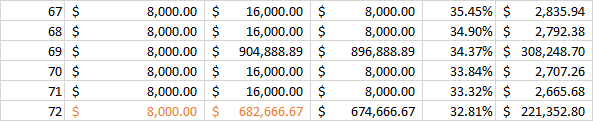We find the present worth of the incremental difference as a function of $i$ and equate it to 0. This allows us to isolate for $i$ and find the monthly nominal interest of 1.56%. Using identical computation as before, we find the effective annual interest, AKA $\Delta$IRR of $\boxed{20.41\%}$. Sinc e the $\Delta$IRR is greater than the MARR. Our economic criterion states that we should go with the higher costing alternative, which is job 2. #### Line of Credit In this situation, we will employ the modified internal rate of interest (MIRR) technique. Since we have chosen job 2, we will use the cash flow presented from previous section. 1. We separate the cashflow that is negative or positive based on net expense or income 2. The expense is under influence of loan interest (0.53% monthly). We find the present value of$3.107M.
3. The income is under influence of investment interest (0.81% monthly). We find the future value of \$4.211M.
4. The MIRR is adjusted such that PW = FW. The formula is:

Finally, MIRR is solved to be $\boxed{10.66\%}$.